Contents | Prev | Next | Index The JavaTM Virtual Machine Specification

A B C D F G I J L M N P R S T W

## l2d

Operation

Convert `long` to `double`

Format

 l2d

Forms

l2d = 138 (0x8a)

Operand Stack

..., value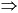..., result

Description

The value on the top of the operand stack must be of type `long`. It is popped from the operand stack and converted to a `double` result using IEEE 754 round to nearest mode. The result is pushed onto the operand stack.

Notes

The l2d instruction performs a widening primitive conversion (§2.6.2) that may lose precision because values of type `double` have only 53 significand bits.

## l2f

Operation

Convert `long` to `float`

Format

 l2f

Forms

l2f = 137 (0x89)

Operand Stack

..., value..., result

Description

The value on the top of the operand stack must be of type `long`. It is popped from the operand stack and converted to a `float` result using IEEE 754 round to nearest mode. The result is pushed onto the operand stack.

Notes

The l2f instruction performs a widening primitive conversion (§2.6.2) that may lose precision because values of type `float` have only 24 significand bits.

## l2i

Operation

Convert `long` to `int`

Format

 l2i

Forms

l2i = 136 (0x88)

Operand Stack

..., value..., result

Description

The value on the top of the operand stack must be of type `long`. It is popped from the operand stack and converted to an `int` result by taking the low-order 32 bits of the `long` value and discarding the high-order 32 bits. The result is pushed onto the operand stack.

Notes

The l2i instruction performs a narrowing primitive conversion (§2.6.3). It may lose information about the overall magnitude of value. The result may also not have the same sign as value.

Operation

Add `long`

Format

Forms

Operand Stack

..., value1, value2..., result

Description

Both value1 and value2 must be of type `long`. The values are popped from the operand stack. The `long` result is value1 + value2. The result is pushed onto the operand stack.

The result is the 64 low-order bits of the true mathematical result in a sufficiently wide two's-complement format, represented as a value of type `long`. If overflow occurs, the sign of the result may not be the same as the sign of the mathematical sum of the two values.

Despite the fact that overflow may occur, execution of an ladd instruction never throws a runtime exception.

Operation

Load `long` from array

Format

Forms

Operand Stack

..., arrayref, index..., value

Description

The arrayref must be of type `reference` and must refer to an array whose components are of type `long`. The index must be of type `int`. Both arrayref and index are popped from the operand stack. The `long` value in the component of the array at index is retrieved and pushed onto the operand stack.

Runtime Exceptions

If arrayref is `null`, laload throws a `NullPointerException`.

Otherwise, if index is not within the bounds of the array referenced by arrayref, the laload instruction throws an `ArrayIndexOutOfBoundsException`.

## land

Operation

Boolean AND `long`

Format

 land

Forms

land = 127 (0x7f)

Operand Stack

..., value1, value2..., result

Description

Both value1 and value2 must be of type `long`. They are popped from the operand stack. A `long` result is calculated by taking the bitwise AND of value1 and value2. The result is pushed onto the operand stack.

## lastore

Operation

Store into `long` array

Format

 lastore

Forms

lastore = 80 (0x50)

Operand Stack

..., arrayref, index, value...

Description

The arrayref must be of type `reference` and must refer to an array whose components are of type `long`. The index must be of type `int`, and value must be of type `long`. The arrayref, index, and value are popped from the operand stack. The `long` value is stored as the component of the array indexed by index.

Runtime Exceptions

If arrayref is `null`, lastore throws a `NullPointerException`.

Otherwise, if index is not within the bounds of the array referenced by arrayref, the lastore instruction throws an `ArrayIndexOutOfBoundsException`.

## lcmp

Operation

Compare `long`

Format

 lcmp

Forms

lcmp = 148 (0x94)

Operand Stack

..., value1, value2..., result

Description

Both value1 and value2 must be of type `long`. They are both popped from the operand stack, and a signed integer comparison is performed. If value1 is greater than value2, the `int` value 1 is pushed onto the operand stack. If value1 is equal to value2, the `int` value 0 is pushed onto the operand stack. If value1 is less than value2, the `int` value -1 is pushed onto the operand stack.

## lconst_<l>

Operation

Push `long` constant

Format

 lconst_

Forms

lconst_0 = 9 (0x9) lconst_1 = 10 (0xa)

Operand Stack

......, <l>

Description

Push the `long` constant <l> (0 or 1) onto the operand stack.

## ldc

Operation

Push item from runtime constant pool

Format

 ldc index

Forms

ldc = 18 (0x12)

Operand Stack

......, value

Description

The index is an unsigned byte that must be a valid index into the runtime constant pool of the current class (§3.6). The runtime constant pool entry at index either must be a runtime constant of type `int` or `float`, or must be a symbolic reference to a string literal (§5.1).

If the runtime constant pool entry is a runtime constant of type `int` or `float`, the numeric value of that runtime constant is pushed onto the operand stack as an `int` or `float`, respectively.

Otherwise, the runtime constant pool entry must be a reference to an instance of class `String` representing a string literal (§5.1). A `reference` to that instance, value, is pushed onto the operand stack.

Notes

The ldc instruction can only be used to push a value of type `float` taken from the float value set (§3.3.2) because a constant of type `float` in the constant pool (§4.4.4) must be taken from the float value set.

## ldc_w

Operation

Push item from runtime constant pool (wide index)

Format

 ldc_w indexbyte1 indexbyte2

Forms

ldc_w = 19 (0x13)

Operand Stack

......, value

Description

The unsigned indexbyte1 and indexbyte2 are assembled into an unsigned 16-bit index into the runtime constant pool of the current class (§3.6), where the value of the index is calculated as (indexbyte1 << 8) | indexbyte2. The index must be a valid index into the runtime constant pool of the current class. The runtime constant pool entry at the index either must be a runtime constant of type `int` or `float`, or must be a symbolic reference to a string literal (§5.1).

If the runtime constant pool entry is a runtime constant of type `int` or `float`, the numeric value of that runtime constant is pushed onto the operand stack as an `int` or `float`, respectively.

Otherwise, the runtime constant pool entry must be a reference to an instance of class `String` representing a string literal (§5.1). A `reference` to that instance, value, is pushed onto the operand stack.

Notes

The ldc_w instruction is identical to the ldc instruction except for its wider runtime constant pool index.

The ldc_w instruction can only be used to push a value of type `float` taken from the float value set (§3.3.2) because a constant of type `float` in the constant pool (§4.4.4) must be taken from the float value set.

## ldc2_w

Operation

Push `long` or `double` from runtime constant pool (wide index)

Format

 ldc2_w indexbyte1 indexbyte2

Forms

ldc2_w = 20 (0x14)

Operand Stack

......, value

Description

The unsigned indexbyte1 and indexbyte2 are assembled into an unsigned 16-bit index into the runtime constant pool of the current class (§3.6), where the value of the index is calculated as (indexbyte1 << 8) | indexbyte2. The index must be a valid index into the runtime constant pool of the current class. The runtime constant pool entry at the index must be a runtime constant of type `long` or `double` (§5.1). The numeric value of that runtime constant is pushed onto the operand stack as a `long` or `double`, respectively.

Notes

Only a wide-index version of the ldc2_w instruction exists; there is no ldc2 instruction that pushes a `long` or `double` with a single-byte index.

The ldc2_w instruction can only be used to push a value of type `double` taken from the double value set (§3.3.2) because a constant of type `double` in the constant pool (§4.4.5) must be taken from the double value set.

## ldiv

Operation

Divide `long`

Format

 ldiv

Forms

ldiv = 109 (0x6d)

Operand Stack

..., value1, value2..., result

Description

Both value1 and value2 must be of type `long`. The values are popped from the operand stack. The `long` result is the value of the Java programming language expression value1 / value2. The result is pushed onto the operand stack.

A `long` division rounds towards 0; that is, the quotient produced for `long` values in n / d is a `long` value q whose magnitude is as large as possible while satisfying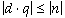. Moreover, q is positive when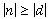and n and d have the same sign, but q is negative when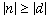and n and d have opposite signs.

There is one special case that does not satisfy this rule: if the dividend is the negative integer of largest possible magnitude for the `long` type and the divisor is -`1`, then overflow occurs and the result is equal to the dividend; despite the overflow, no exception is thrown in this case.

Runtime Exception

If the value of the divisor in a `long` division is 0, ldiv throws an `ArithmeticException`.

Operation

Load `long` from local variable

Format

Forms

Operand Stack

......, value

Description

The index is an unsigned byte. Both index and index + 1 must be indices into the local variable array of the current frame (§3.6). The local variable at index must contain a `long`. The value of the local variable at index is pushed onto the operand stack.

Notes

The lload opcode can be used in conjunction with the wide instruction to access a local variable using a two-byte unsigned index.

Operation

Load `long` from local variable

Format

Forms

Operand Stack

......, value

Description

Both <n> and <n> + 1 must be indices into the local variable array of the current frame (§3.6). The local variable at <n> must contain a `long`. The value of the local variable at <n> is pushed onto the operand stack.

Notes

Each of the lload_<n> instructions is the same as lload with an index of <n>, except that the operand <n> is implicit.

## lmul

Operation

Multiply `long`

Format

 lmul

Forms

lmul = 105 (0x69)

Operand Stack

..., value1, value2..., result

Description

Both value1 and value2 must be of type `long`. The values are popped from the operand stack. The `long` result is value1 * value2. The result is pushed onto the operand stack.

The result is the 64 low-order bits of the true mathematical result in a sufficiently wide two's-complement format, represented as a value of type `long`. If overflow occurs, the sign of the result may not be the same as the sign of the mathematical sum of the two values.

Despite the fact that overflow may occur, execution of an lmul instruction never throws a runtime exception.

## lneg

Operation

Negate `long`

Format

 lneg

Forms

lneg = 117 (0x75)

Operand Stack

..., value..., result

Description

The value must be of type `long`. It is popped from the operand stack. The `long` result is the arithmetic negation of value, -value. The result is pushed onto the operand stack.

For `long` values, negation is the same as subtraction from zero. Because the Java virtual machine uses two's-complement representation for integers and the range of two's-complement values is not symmetric, the negation of the maximum negative `long` results in that same maximum negative number. Despite the fact that overflow has occurred, no exception is thrown.

For all `long` values `x`, -`x` equals (~`x`) + `1`.

## lookupswitch

Operation

Access jump table by key match and jump

Format

 lookupswitch <0-3 byte pad\> defaultbyte1 defaultbyte2 defaultbyte3 defaultbyte4 npairs1 npairs2 npairs3 npairs4 match-offset pairs...

Forms

lookupswitch = 171 (0xab)

Operand Stack

..., key...

Description

A lookupswitch is a variable-length instruction. Immediately after the lookupswitch opcode, between zero and three null bytes (zeroed bytes, not the null object) are inserted as padding. The number of null bytes is chosen so that the defaultbyte1 begins at an address that is a multiple of four bytes from the start of the current method (the opcode of its first instruction). Immediately after the padding follow a series of signed 32-bit values: default, npairs, and then npairs pairs of signed 32-bit values. The npairs must be greater than or equal to 0. Each of the npairs pairs consists of an `int` match and a signed 32-bit offset. Each of these signed 32-bit values is constructed from four unsigned bytes as (byte1 << 24) | (byte2 << 16) | (byte3 << 8) | byte4.

The table match-offset pairs of the lookupswitch instruction must be sorted in increasing numerical order by match.

The key must be of type `int` and is popped from the operand stack. The key is compared against the match values. If it is equal to one of them, then a target address is calculated by adding the corresponding offset to the address of the opcode of this lookupswitch instruction. If the key does not match any of the match values, the target address is calculated by adding default to the address of the opcode of this lookupswitch instruction. Execution then continues at the target address.

The target address that can be calculated from the offset of each match-offset pair, as well as the one calculated from default, must be the address of an opcode of an instruction within the method that contains this lookupswitch instruction.

Notes

The alignment required of the 4-byte operands of the lookupswitch instruction guarantees 4-byte alignment of those operands if and only if the method that contains the lookupswitch is positioned on a 4-byte boundary.

The match-offset pairs are sorted to support lookup routines that are quicker than linear search.

## lor

Operation

Boolean OR `long`

Format

 lor

Forms

lor = 129 (0x81)

Operand Stack

..., value1, value2..., result

Description

Both value1 and value2 must be of type `long`. They are popped from the operand stack. A `long` result is calculated by taking the bitwise inclusive OR of value1 and value2. The result is pushed onto the operand stack.

## lrem

Operation

Remainder `long`

Format

 lrem

Forms

lrem = 113 (0x71)

Operand Stack

..., value1, value2..., result

Description

Both value1 and value2 must be of type `long`. The values are popped from the operand stack. The `long` result is value1 - (value1 / value2) * value2. The result is pushed onto the operand stack.

The result of the lrem instruction is such that (`a`/`b`)*`b` + (`a%b`) is equal to `a`. This identity holds even in the special case in which the dividend is the negative `long` of largest possible magnitude for its type and the divisor is -`1` (the remainder is `0`). It follows from this rule that the result of the remainder operation can be negative only if the dividend is negative and can be positive only if the dividend is positive; moreover, the magnitude of the result is always less than the magnitude of the divisor.

Runtime Exception

If the value of the divisor for a `long` remainder operator is `0`, lrem throws an `ArithmeticException`.

## lreturn

Operation

Return `long` from method

Format

 lreturn

Forms

Operand Stack

..., value[empty]

Description

The current method must have return type `long`. The value must be of type `long`. If the current method is a `synchronized` method, the monitor acquired or reentered on invocation of the method is released or exited (respectively) as if by execution of a monitorexit instruction. If no exception is thrown, value is popped from the operand stack of the current frame (§3.6) and pushed onto the operand stack of the frame of the invoker. Any other values on the operand stack of the current method are discarded.

The interpreter then returns control to the invoker of the method, reinstating the frame of the invoker.

Runtime Exceptions

If the current method is a `synchronized` method and the current thread is not the owner of the monitor acquired or reentered on invocation of the method, lreturn throws an `IllegalMonitorStateException`. This can happen, for example, if a `synchronized` method contains a monitorexit instruction, but no monitorenter instruction, on the object on which the method is synchronized.

Otherwise, if the virtual machine implementation enforces the rules on structured use of locks described in Section 8.13 and if the first of those rules is violated during invocation of the current method, then lreturn throws an `IllegalMonitorStateException`.

## lshl

Operation

Shift left

Format

 lshl

`long`

Forms

lshl = 121 (0x79)

Operand Stack

..., value1, value2..., result

Description

The value1 must be of type `long`, and value2 must be of type `int`. The values are popped from the operand stack. A `long` result is calculated by shifting value1 left by s bit positions, where s is the low 6 bits of value2. The result is pushed onto the operand stack.

Notes

This is equivalent (even if overflow occurs) to multiplication by 2 to the power s. The shift distance actually used is therefore always in the range 0 to 63, inclusive, as if value2 were subjected to a bitwise logical AND with the mask value 0x3f.

## lshr

Operation

Arithmetic shift right `long`

Format

 lshr

Forms

lshr = 123 (0x7b)

Operand Stack

..., value1, value2..., result

Description

The value1 must be of type `long`, and value2 must be of type `int`. The values are popped from the operand stack. A `long` result is calculated by shifting value1 right by s bit positions, with sign extension, where s is the value of the low 6 bits of value2. The result is pushed onto the operand stack.

Notes

The resulting value is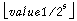, where s is value2 & 0x3f. For nonnegative value1, this is equivalent to truncating `long` division by 2 to the power `s`. The shift distance actually used is therefore always in the range 0 to 63, inclusive, as if value2 were subjected to a bitwise logical AND with the mask value 0x3f.

## lstore

Operation

Store `long` into local variable

Format

 lstore index

Forms

lstore = 55 (0x37)

Operand Stack

..., value...

Description

The index is an unsigned byte. Both index and index + 1 must be indices into the local variable array of the current frame (§3.6). The value on the top of the operand stack must be of type `long`. It is popped from the operand stack, and the local variables at index and index + 1 are set to value.

Notes

The lstore opcode can be used in conjunction with the wide instruction to access a local variable using a two-byte unsigned index.

## lstore_<n>

Operation

Store `long` into local variable

Format

 lstore_

Forms

lstore_0 = 63 (0x3f) lstore_1 = 64 (0x40) lstore_2 = 65 (0x41) lstore_3 = 66 (0x42)

Operand Stack

..., value...

Description

Both <n> and <n> + 1 must be indices into the local variable array of the current frame (§3.6). The value on the top of the operand stack must be of type `long`. It is popped from the operand stack, and the local variables at <n> and <n> + 1 are set to value.

Notes

Each of the lstore_<n> instructions is the same as lstore with an index of <n>, except that the operand <n> is implicit.

## lsub

Operation

Subtract `long`

Format

 lsub

Forms

lsub = 101 (0x65)

Operand Stack

..., value1, value2..., result

Description

Both value1 and value2 must be of type `long`. The values are popped from the operand stack. The `long` result is value1 - value2. The result is pushed onto the operand stack.

For `long` subtraction, `a`-`b` produces the same result as `a`+`(`-`b)`. For `long` values, subtraction from zero is the same as negation.

The result is the 64 low-order bits of the true mathematical result in a sufficiently wide two's-complement format, represented as a value of type `long`. If overflow occurs, then the sign of the result may not be the same as the sign of the mathematical sum of the two values.

Despite the fact that overflow may occur, execution of an lsub instruction never throws a runtime exception.

## lushr

Operation

Logical shift right `long`

Format

 lushr

Forms

lushr = 125 (0x7d)

Operand Stack

..., value1, value2..., result

Description

The value1 must be of type `long`, and value2 must be of type `int`. The values are popped from the operand stack. A `long` result is calculated by shifting value1 right logically (with zero extension) by the amount indicated by the low 6 bits of value2. The result is pushed onto the operand stack.

Notes

If value1 is positive and s is value2 & 0x3f, the result is the same as that of value1 >> s; if value1 is negative, the result is equal to the value of the expression (value1 >> s) + (2L << ~s). The addition of the (2L << ~s) term cancels out the propagated sign bit. The shift distance actually used is always in the range 0 to 63, inclusive.

## lxor

Operation

Boolean XOR `long`

Format

 lxor

Forms

lxor = 131 (0x83)

Operand Stack

..., value1, value2..., result

Description

Both value1 and value2 must be of type `long`. They are popped from the operand stack. A `long` result is calculated by taking the bitwise exclusive OR of value1 and value2. The result is pushed onto the operand stack.

The JavaTM Virtual Machine Specification